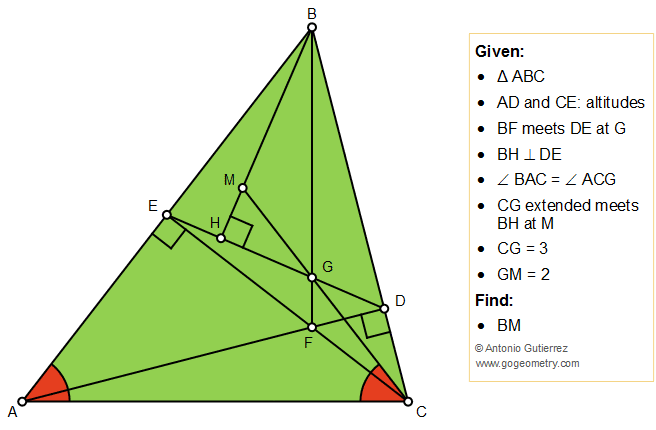## Tuesday, July 30, 2019

### Geometry Problem 1442 Triangle, Altitudes, Orthocenter, Perpendicular, Congruent Angles, Similarity, Measurement, iPad apps

Geometry Problem. Post your solution in the comment box below.
Level: Mathematics Education, High School, Honors Geometry, College.

Details: Click on the figure below.1.Since < BDE = A, < MBG = (90-A) - (90-C) = C-A = < MCB

It follows that BM^2 = MG.MC = 10
So BM = sqrt10

Sumith Peiris
Moratuwa
Sri Lanka

2.Nice solution, Sumith.

1.Thank u Ajit